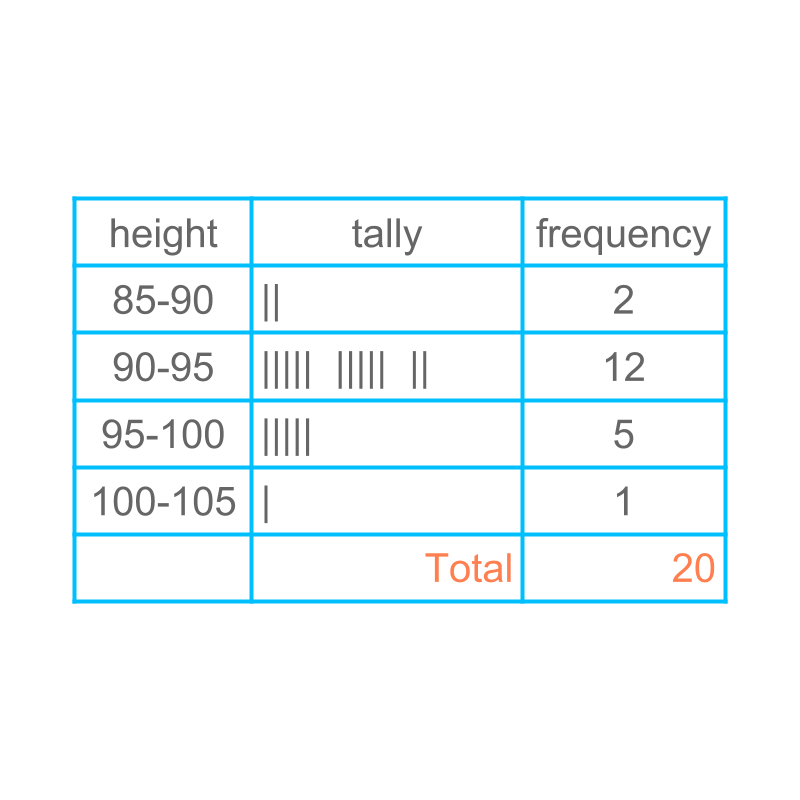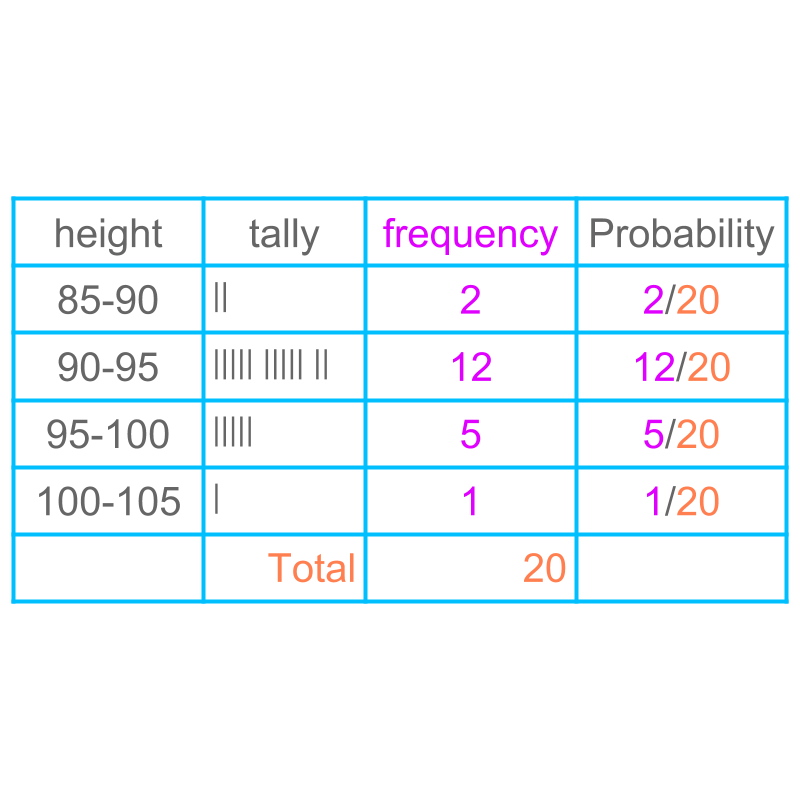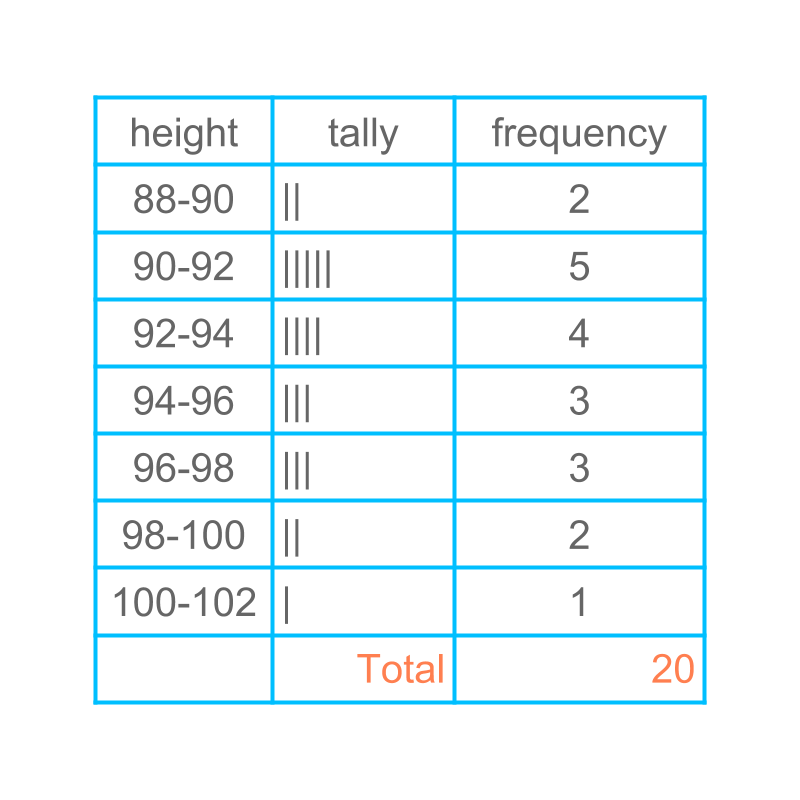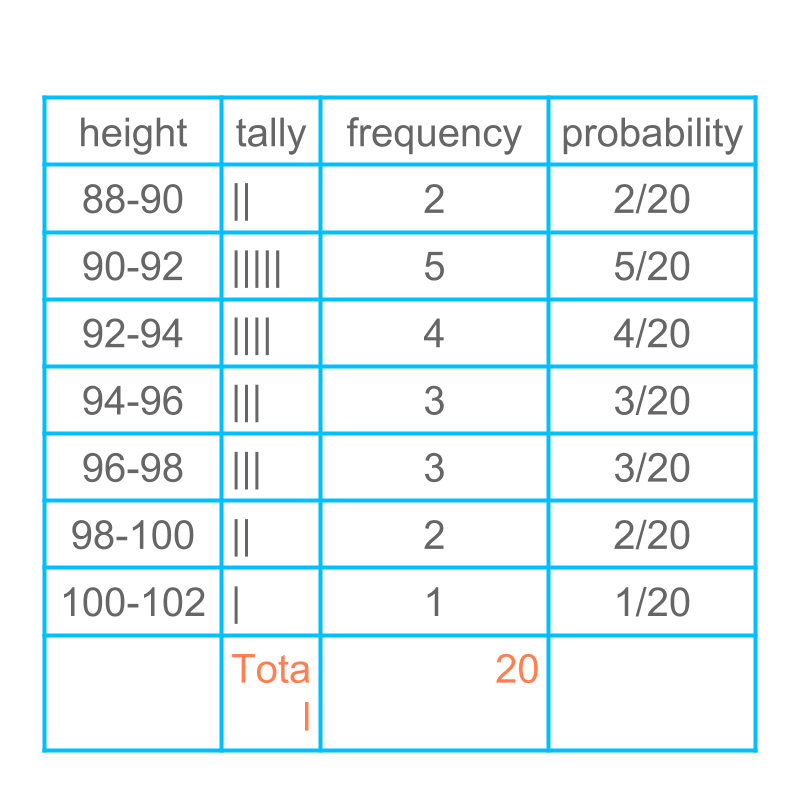maths > statistics-basics

Predicting using Grouped Data : Probability

what you'll learn...

overview

In this page, finding probability of grouped data is explained with some examples.

predicting

Consider the data height of students in a class of $20$$20$ students.

$90.2$$90.2$, $97.4$$97.4$, $99.7$$99.7$, $88.3$$88.3$, $90.7$$90.7$

$93.5$$93.5$, $96.8$$96.8$, $89.5$$89.5$, $99.5$$99.5$, $98.9$$98.9$

$91.2$$91.2$, $101.3$$101.3$, $94.0$$94.0$, $93.4$$93.4$, $93.1$$93.1$

$94.7$$94.7$, $96.9$$96.9$, $92.8$$92.8$, $91.8$$91.8$, $99.6$$99.6$

The figure shows the grouped data with ranges less than $90$$90$, $90$$90$ to $95$$95$ range, $95$$95$ to $100$$100$ range, and greater than $100$$100$.Considering this to be representative data for students of that age group, We try to predict the height of a student.

The probability of a random student in the height range $95$$95$ to $100$$100$ $=5/20$$= 5 / 20$The probability of a random student of that age group in each of the range is given in the table.

zero probability

The probability of a random student to be exactly $97$$97$cm high is 0. It is noted that the probability of getting one particular value is $0$$0$ in continuous distribution. This will be explained in detail in higher classes.

different representation

Consider the data height of students in a class of $20$$20$ students.

$90.2$$90.2$, $97.4$$97.4$, $99.7$$99.7$, $88.3$$88.3$, $90.7$$90.7$

$93.5$$93.5$, $96.8$$96.8$, $89.5$$89.5$, $99.5$$99.5$, $98.9$$98.9$

$91.2$$91.2$, $101.3$$101.3$, $94.0$$94.0$, $93.4$$93.4$, $93.1$$93.1$

$94.7$$94.7$, $96.9$$96.9$, $92.8$$92.8$, $91.8$$91.8$, $99.6$$99.6$

The figure shows the grouped data with ranges less than $90$$90$, $90$$90$ to $95$$95$ range, $95$$95$ to $100$$100$ range, and greater than $100$$100$.

It is noted that, This is one possible grouping and the data can be grouped in different ranges.A dirrerent grouping is applied and the data is provided in the table.Probability of the same is given in the figure.

summary

Probability in Grouped Data : Probability is computed for of a range of values in grouped data. Probability of a range equals, the number of occurances in the range, divided by, the number of occurances in the data.

Outline